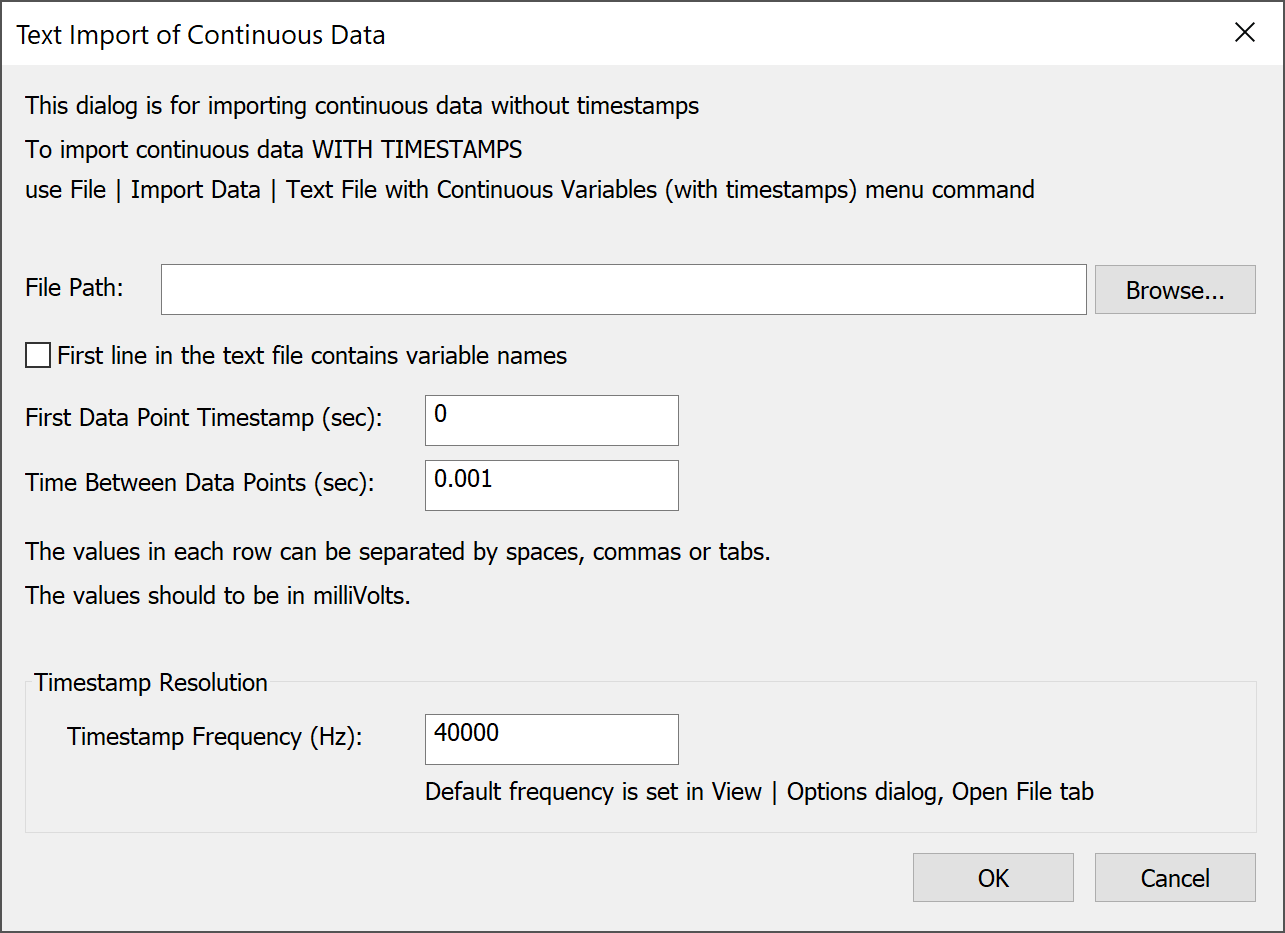# Importing Continuous Data From Text Files

NeuroExplorer can import continuous data from a multicolumn text file where each column corresponds to a continuous variable. Here is an example:

```ContChannel1    ContChannel2
114.74609       -63.47656
56.15234        -358.88672
-187.98828      -63.47656
-48.82813       388.18359
-26.85547       285.64453
```

Use File | Import Data | Text File with Continuous Variables (no timestamps)… menu command to import such a data file. The following dialog will be shown:• First line contains variable names - if this option is selected, the fields of the first line of the text file are used as variable names. The second line in the file is the first row of data.

• First Data Point Timestamp specifies the timestamp of the first data point in each continuous variable.

• Time Between Data Points specifies the time step used in calculation of the timestamp for each row of data. For data row n (n = 1, 2, …) in the text file, the data row timestamp is calculated using the following formula:

```DataRowTimestamp = FirstDataPoint + (n-1)*TimeBetweenDataPoints
```

If you have a text file with the timestamps in the first column of the file:

```Time      ContChannel01
0.000400  114.746094
0.000500  56.152344
0.000600  -187.988281
0.000700  -48.828125
0.000800  -26.855469
```

use File | Import Data | Text File with Continuous Variables (with timestamps)… menu command to import such a data file.